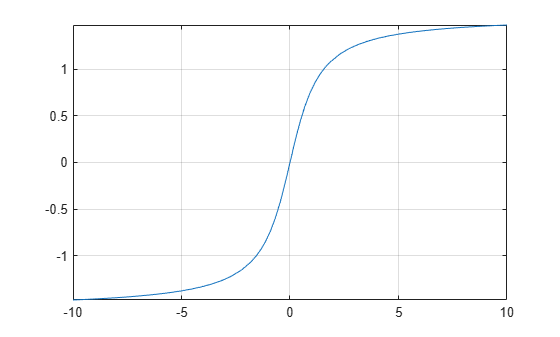# atan

Symbolic inverse tangent function

## Syntax

``atan(X)``

## Description

example

````atan(X)` returns the inverse tangent function (arctangent function) of `X`. All angles are in radians. For real values of `X`, `atan(X)` returns values in the interval `[-pi/2,pi/2]`.For complex values of `X`, `atan(X)` returns complex values with the real parts in the interval `[-pi/2,pi/2]`. ```

## Examples

### Inverse Tangent Function for Numeric and Symbolic Arguments

Depending on its arguments, `atan` returns floating-point or exact symbolic results.

Compute the inverse tangent function for these numbers. Because these numbers are not symbolic objects, `atan` returns floating-point results.

`A = atan([-1, -1/3, -1/sqrt(3), 1/2, 1, sqrt(3)])`
```A = -0.7854 -0.3218 -0.5236 0.4636 0.7854 1.0472```

Compute the inverse tangent function for the numbers converted to symbolic objects. For many symbolic (exact) numbers, `atan` returns unresolved symbolic calls.

`symA = atan(sym([-1, -1/3, -1/sqrt(3), 1/2, 1, sqrt(3)]))`
```symA = [ -pi/4, -atan(1/3), -pi/6, atan(1/2), pi/4, pi/3]```

Use `vpa` to approximate symbolic results with floating-point numbers:

`vpa(symA)`
```ans = [ -0.78539816339744830961566084581988,... -0.32175055439664219340140461435866,... -0.52359877559829887307710723054658,... 0.46364760900080611621425623146121,... 0.78539816339744830961566084581988,... 1.0471975511965977461542144610932]```

### Plot Inverse Tangent Function

Plot the inverse tangent function on the interval from -10 to 10.

```syms x fplot(atan(x),[-10 10]) grid on```### Handle Expressions Containing Inverse Tangent Function

Many functions, such as `diff`, `int`, `taylor`, and `rewrite`, can handle expressions containing `atan`.

Find the first and second derivatives of the inverse tangent function:

```syms x diff(atan(x), x) diff(atan(x), x, x)```
```ans = 1/(x^2 + 1) ans = -(2*x)/(x^2 + 1)^2```

Find the indefinite integral of the inverse tangent function:

`int(atan(x), x)`
```ans = x*atan(x) - log(x^2 + 1)/2```

Find the Taylor series expansion of `atan(x)`:

`taylor(atan(x), x)`
```ans = x^5/5 - x^3/3 + x```

Rewrite the inverse tangent function in terms of the natural logarithm:

`rewrite(atan(x), 'log')`
```ans = (log(1 - x*1i)*1i)/2 - (log(1 + x*1i)*1i)/2```

## Input Arguments

collapse all

Input, specified as a symbolic number, variable, expression, or function, or as a vector or matrix of symbolic numbers, variables, expressions, or functions.